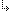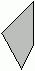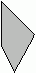- 4.37.2.20. -4. Standard Units4.37. Graph - Graph unit4.37.2. Graph Unit Procedures and Functions4.37.2.20. FillPoly procedure

## 4.37.2.20. FillPoly procedure

Targets: MS-DOS, Win32

Graph Unit

Fills a polygon, using the scan converter

Declaration:
`procedure FillPoly(NumVert: DWORD; var Vert);`
Remarks:
Vert is an untyped parameter that contains the coordinates of each vertex in the polygon. NumVert specifies the number of coordinate pairs in Vert. A coordinate pair consists of two Longint values, an X and a Y value. FillPoly calculates all the horizontal intersections, and then fills it using the current fill style and color defined by SetFillColor, SetFillPattern or SetFillStyle. This function is different from Borland's GRAPH unit. Polygons must have angles less than 180 degrees. That is, they must be convex.2) Invalid polygon

If you want to draw polygon (2), you must split it up into two valid polygons:+=- 4.37.2.20. -